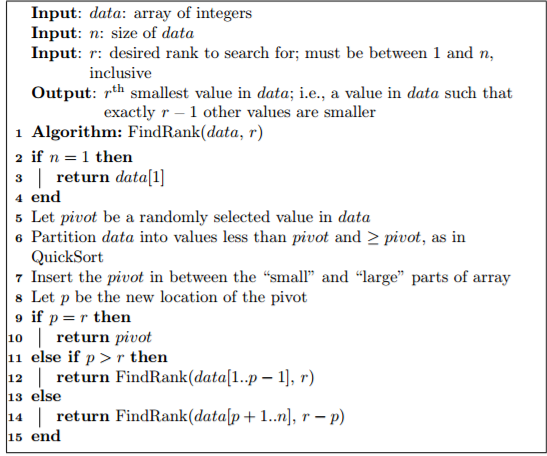# Answered! Input: data: array of integers Input n: size of data Input r: desired rank to search for: must be between 1 and n…

Consider the algorithm below, which accepts an array data and an integer r and returns the rth smallest value in the arrayDon't use plagiarized sources. Get Your Custom Essay on
Answered! Input: data: array of integers Input n: size of data Input r: desired rank to search for: must be between 1 and n…
GET AN ESSAY WRITTEN FOR YOU FROM AS LOW AS \$13/PAGE

1. Prove that r − p must be between 1 and the length of data[p + 1..n], inclusive, when line 14 is executed.

2. Prove that this algorithm returns the r th smallest value in the array. You may assume that all elements of data are unique

Input: data: array of integers Input n: size of data Input r: desired rank to search for: must be between 1 and n inclusive output rth smallest value in data; i.e., a value in data such that exactly r 1 other values are smaller 1 Algorithm: FindRank(data, r) 2 if n 1 then 3 I return data 4 end 5 Let pivot be a randomly selected value in data 6 Partition data into values less than pivot and pivot, as in Quick Sort 7 Insert the pivot in between the “small” and “large” parts of array 8 Let p be the new location of the pivot 9 if r then 10 return pivot 11 else if p r then 12 I return FindRank data 1.p -1], r) 13 else 14 return FindRank (data p 1…n] r p) 15 end

Let us consider that the code works exactly as mentioned till line 8.

So we have three possible cases:
p = r
p > r
p < r

anyway
let us consider question 1.
when we execute line 14, we have determined that p < r. (doubts?)
if (p < r), r – p must be posiive or r-p >= 1.

we know that data[1 .. n] have n elements, so data[p+1 .. n] have n – p elements. For proper operations, r <= n

r <= n
or r-p <= n-p (proved)

for question 2
if p = r we know that there are p items before the ‘pivot’, so it is obhious that pivot is the rth smallest item no doubt.

if p > r, we are sure that pivot is larger than the item we are searching. We also know that at line 8, sub array 1…p-1 have elements strictly less than pivot. Under all these circumstances, the item we are searching for is present in the array[1 .. p-1] and rank of that item in the sub array is equal to the rank of the item in the main array. So the recusrive call is valid.

if p < r, we are sure that pivot is smaller than the item we are searching. We also know that at line 8, sub array p+1…n have elements strictly larger than pivot. since r > p, i item we are finding is present in this sub array, but the rank of the item will be different. the rank would be r – p (since p number of items which were smaller are no more present here). so the reccursive call here is valid too.